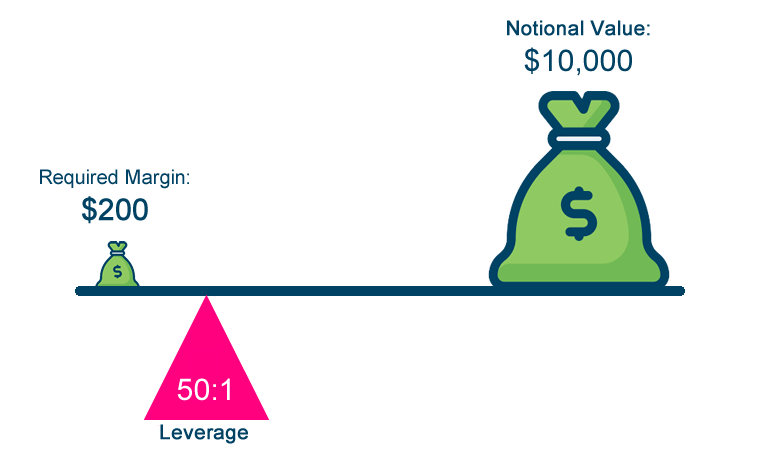# The Relationship Between Margin and Leverage

What is the relationship between Margin and Leverage?

You use margin to create leverage.

Leverage is the increased “trading power” that is available when using a margin account.

Leverage is expressed as a ratio.

Leverage is the ratio between the amount of money you really have and the amount of money you can trade.

It is usually expressed with an “X:1” format.

For example, if you wanted to trade 1 standard lot of USD/JPY without margin, you would need \$100,000 in your account.

But with a Margin Requirement of just 1%, you would only have to deposit \$1,000 in your account.

The leverage provided for this trade would be 100:1.

Here are examples of Leverage Ratios depending on the Margin Requirement:

 Currency Pair Margin Requirement Leverage Ratio EUR/USD 2% 50:1 GBP/USD 5% 20:1 USD/JPY 4% 25:1 EUR/AUD 3% 33:1

Here’s how to calculate Leverage:

`Leverage = 1 / Margin Requirement`

For example, if the Margin Requirement is 2%, here’s how to calculate leverage:

`50 = 1 / .02`

The leverage is 50, which is expressed as a ratio, 50:1

Here’s how to calculate the Margin Requirement based on the Leverage Ratio:

`Margin Requirement = 1 / Leverage Ratio`

For example, if the Leverage Ratio is 100:1, here’s how to calculate the Margin Requirement.

`0.01 = 1 / 100`

The Margin Requirement is 0.01 or 1%.

As you can see, leverage has an inverse relationship to margin.

“Leverage” and “margin” refer to the same concept, just from a slightly different angle.

When a trader opens a position, they are required to put up a fraction of that position’s value “in good faith”. In this case, the trader is said to be “leveraged”.

The “fraction” part which is expressed in percentage terms is known as the “Margin Requirement”. For example, 2%.

The actual amount that is required to be put up is known as the “Required Margin”.

For example, 2% of a \$100,000 position size would be \$2,000.

The \$2,000 is the Required Margin to open this specific position.

Since you are able to trade a \$100,000 position size with just \$2,000, your leverage ratio is 50:1.

```Leverage = 1 /Margin Requirement

50 = 1 / 0.02```Forex Margin vs. Securities Margin

Forex margin and securities margin are two very different things. Understanding the difference is important.

In the securities world, margin is the money you borrow as a partial down payment, usually up to 50% of the purchase price, to buy and own a stock, bond, or ETF.

This practice is often referred to as “buying on margin”.

So if you’re trading stocks on margin, you’re borrowing money from your stock broker to purchase stock. Basically, a loan from the brokerage firm.

In the forex market, margin is the amount of money that you must deposit and keep on hand with your trading platform when you open a position.

It is NOT a down payment and you do NOT own the underlying currency pair.

Margin can be looked at as a good faith deposit or collateral that’s used to ensure each party (buyer and seller) can meet their obligations of the agreement.

Unlike margin in stock trading, margin in forex trading is not borrowed money.

When trading forex, nothing is actually being bought or sold, only the agreement (or contract) to buy or sell are exchanged, so borrowing is not needed.

The term “margin” is used across multiple financial markets. However, there is a difference between how margin is used when trading securities versus when trading forex. Understanding this difference is essential prior to trading forex.

0 replies